# Möbius strip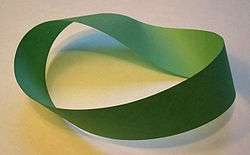A Möbius strip made with a piece of paper and tape. If an ant were to crawl along the length of this strip, it would return to its starting point having traversed the entire length of the strip (on both sides of the original paper) without ever crossing an edge.

The Möbius strip or Möbius band (/ˈmɜːrbiəs/ (non-rhotic) or US /ˈmbiəs/; German: [ˈmøːbi̯ʊs]), Mobius or Moebius, is a surface with only one side and only one boundary. The Möbius strip has the mathematical property of being non-orientable. It can be realized as a ruled surface. It was discovered independently by the German mathematicians August Ferdinand Möbius and Johann Benedict Listing in 1858.

An example of a Möbius strip can be created by taking a paper strip and giving it a half-twist, and then joining the ends of the strip to form a loop. However, the Möbius strip is not a surface of only one exact size and shape, such as the half-twisted paper strip depicted in the illustration. Rather, mathematicians refer to the closed Möbius band as any surface that is homeomorphic to this strip. Its boundary is a simple closed curve, i.e., homeomorphic to a circle. This allows for a very wide variety of geometric versions of the Möbius band as surfaces each having a definite size and shape. For example, any rectangle can be glued to itself (by identifying one edge with the opposite edge after a reversal of orientation) to make a Möbius band. Some of these can be smoothly modeled in Euclidean space, and others cannot.

A half-twist clockwise gives an embedding of the Möbius strip different from that of a half-twist counterclockwise – that is, as an embedded object in Euclidean space the Möbius strip is a chiral object with right- or left-handedness. However, the underlying topological spaces within the Möbius strip are homeomorphic in each case. There are an infinite number of topologically different embeddings of the same topological space into three-dimensional space, as the Möbius strip can also be formed by twisting the strip an odd number of times greater than one, or by knotting and twisting the strip, before joining its ends. The complete open Möbius band is an example of a topological surface that is closely related to the standard Möbius strip but that is not homeomorphic to it.

It is straightforward to find algebraic equations, the solutions of which have the topology of a Möbius strip, but in general these equations do not describe the same geometric shape that one gets from the twisted paper model described above. In particular, the twisted paper model is a developable surface, having zero Gaussian curvature. A system of differential-algebraic equations that describes models of this type was published in 2007 together with its numerical solution.

The Euler characteristic of the Möbius strip is zero.

## Properties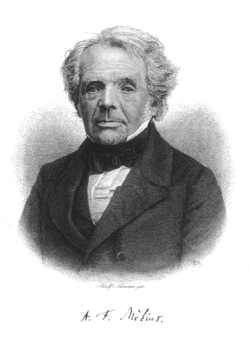August Ferdinand Möbius

The Möbius strip has several curious properties. A line drawn starting from the seam down the middle meets back at the seam but at the other side. If continued the line meets the starting point, and is double the length of the original strip. This single continuous curve demonstrates that the Möbius strip has only one boundary.

Cutting a Möbius strip along the center line with a pair of scissors yields one long strip with two full twists in it, rather than two separate strips; the result is not a Möbius strip. This happens because the original strip only has one edge that is twice as long as the original strip. Cutting creates a second independent edge, half of which was on each side of the scissors. Cutting this new, longer, strip down the middle creates two strips wound around each other, each with two full twists.

If the strip is cut along about a third of the way in from the edge, it creates two strips: One is a thinner Möbius strip – it is the center third of the original strip, comprising 1/3 of the width and the same length as the original strip. The other is a longer but thin strip with two full twists in it – this is a neighborhood of the edge of the original strip, and it comprises 1/3 of the width and twice the length of the original strip.

Other analogous strips can be obtained by similarly joining strips with two or more half-twists in them instead of one. For example, a strip with three half-twists, when divided lengthwise, becomes a strip tied in a trefoil knot. (If this knot is unravelled, the strip is made with eight half-twists in addition to an overhand knot.) A strip with N half-twists, when bisected, becomes a strip with N + 1 full twists. Giving it extra twists and reconnecting the ends produces figures called paradromic rings.

## Geometry and topology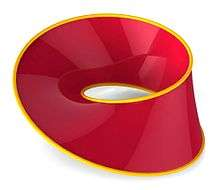A ray-traced parametric plot of a Möbius strip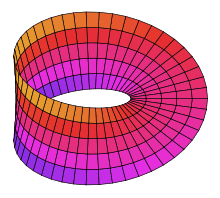A parametric plot of a Möbius strip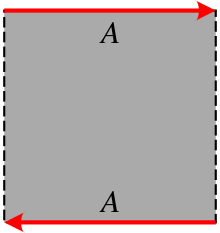To turn a rectangle into a Möbius strip, join the edges labelled A so that the directions of the arrows match.

One way to represent the Möbius strip as a subset of three-dimensional Euclidean space is using the parametrization:where 0 ≤ u < 2π and −1 ≤ v ≤ 1. This creates a Möbius strip of width 1 whose center circle has radius 1, lies in the xy plane and is centered at (0, 0, 0). The parameter u runs around the strip while v moves from one edge to the other.

In cylindrical polar coordinates (r, θ, z), an unbounded version of the Möbius strip can be represented by the equation:### Widest isometric embedding in 3-space

If a smooth Möbius strip in 3-space is a rectangular one – that is, created from identifying two opposite sides of a geometrical rectangle with bending but not stretching the surface – then such an embedding is known to be possible if the aspect ratio of the rectangle is greater than the square root of 3. (Note that it is the shorter sides of the rectangle that are identified to obtain the Möbius strip.) For an aspect ratio less than or equal to the square root of 3, however, a smooth embedding of a rectangular Möbius strip into 3-space may be impossible.

As the aspect ratio approaches the limiting ratio of 3 from above, any such rectangular Möbius strip in 3-space seems to approach a shape that in the limit can be thought of as a strip of three equilateral triangles, folded on top of one another so that they occupy just one equilateral triangle in 3-space.

If the Möbius strip in 3-space is only once continuously differentiable (in symbols: C1), however, then the theorem of Nash-Kuiper shows that there is no lower bound.

A method of making a Möbius strip from a rectangular strip too wide to simply twist and join (e.g., a rectangle only 1 unit long and 1 unit wide) is to first fold the wide direction back and forth using an even number of folds—an accordion fold—so that the folded strip becomes narrow enough that it can be twisted and joined, much as a single long-enough strip can be joined. With two folds, for example, a 1 × 1 strip would become a 1 × ⅓ folded strip whose cross section is in the shape of an 'N' and would remain an 'N' after a half-twist. This folded strip, three times as long as it is wide, would be long enough to then join at the ends. This method works in principle but becomes impractical after sufficiently many folds, if paper is used. Using normal paper, this construction can be folded flat, with all the layers of the paper in a single plane. But mathematically, it is not clear whether this is possible without stretching the surface of the rectangle.

### Topology

Topologically, the Möbius strip can be defined as the square [0, 1] × [0, 1] with its top and bottom sides identified by the relation (x, 0) ~ (1 − x, 1) for 0 ≤ x ≤ 1, as in the diagram on the right.

A less used presentation of the Möbius strip is as the topological quotient of a torus. A torus can be constructed as the square [0, 1] × [0, 1] with the edges identified as (0, y) ~ (1, y) (glue left to right) and (x, 0) ~ (x, 1) (glue bottom to top). If one then also identified (x, y) ~ (y, x), then one obtains the Möbius strip. The diagonal of the square (the points (x, x) where both coordinates agree) becomes the boundary of the Möbius strip, and carries an orbifold structure, which geometrically corresponds to "reflection" – geodesics (straight lines) in the Möbius strip reflect off the edge back into the strip. Notationally, this is written as T2/S2 – the 2-torus quotiented by the group action of the symmetric group on two letters (switching coordinates), and it can be thought of as the configuration space of two unordered points on the circle, possibly the same (the edge corresponds to the points being the same), with the torus corresponding to two ordered points on the circle.

The Möbius strip is a two-dimensional compact manifold (i.e. a surface) with boundary. It is a standard example of a surface that is not orientable. In fact, the Möbius strip is the epitome of the topological phenomenon of nonorientability. This is because 1) two-dimensional shapes (surfaces) are the lowest-dimensional shapes for which nonorientability is possible, and 2) the Möbius strip is the only surface that is topologically a subspace of every non-orientable surface. As a result, any surface is non-orientable if and only if it contains a Möbius band as a subspace.

The Möbius strip is also a standard example used to illustrate the mathematical concept of a fiber bundle. Specifically, it is a nontrivial bundle over the circle S1 with a fiber the unit interval, I = [0, 1]. Looking only at the edge of the Möbius strip gives a nontrivial two point (or Z2) bundle over S1.

### Computer graphics

A simple construction of the Möbius strip that can be used to portray it in computer graphics or modeling packages is as follows :

• Take a rectangular strip. Rotate it around a fixed point not in its plane. At every step also rotate the strip along a line in its plane (the line that divides the strip in two) and perpendicular to the main orbital radius. The surface generated on one complete revolution is the Möbius strip.
• Take a Möbius strip and cut it along the middle of the strip. This forms a new strip, which is a rectangle joined by rotating one end a whole turn. By cutting it down the middle again, this forms two interlocking whole-turn strips.

### Open Möbius band

The open Möbius band is formed by deleting the boundary of the standard Möbius band. It is constructed from the set S = { (x, y) ∈ R2 : 0 ≤ x ≤ 1 and 0 < y < 1 } by identifying (glueing) the points (0, y) and (1, 1 − y) for all 0 < y < 1.

It may be constructed as a surface of constant positive, negative, or zero (Gaussian) curvature. In the cases of negative and zero curvature, the Möbius band can be constructed as a (geodesically) complete surface, which means that all geodesics ("straight lines" on the surface) may be extended indefinitely in either direction.

Constant negative curvature: Like the plane and the open cylinder, the open Möbius band admits not only a complete metric of constant curvature 0, but also a complete metric of constant negative curvature, say −1. One way to see this is to begin with the upper half plane (Poincaré) model of the hyperbolic plane ℍ, namely ℍ = { (x, y) ∈ ℝ2 | y > 0} with the Riemannian metric given by (dx2 + dy2) / y2. The orientation-preserving isometries of this metric are all the maps f : ℍ → ℍ of the form f(z) := (az + b) / (cz + d), where a, b, c, d are real numbers satisfying adbc = 1. Here z is a complex number with Im(z) > 0, and we have identified ℍ with {z ∈ ℂ | Im(z) > 0} endowed with the Riemannian metric that was mentioned. Then one orientation-reversing isometry g of ℍ given by g(z) := -conj(z), where conj(z) denotes the complex conjugate of z. These facts imply that the mapping h : ℍ → ℍ given by h(z) := −2⋅conj(z) is an orientation-reversing isometry of ℍ that generates an infinite cyclic group G of isometries. (Its square is the isometry h(z) := 4⋅z, which can be expressed as (2z + 0) / (0z + 1/2).) The quotient ℍ / G of the action of this group can easily be seen to be topologically a Möbius band. But it is also easy to verify that it is complete and non-compact, with constant negative curvature −1.

The group of isometries of this Möbius band is 1-dimensional and is isomorphic to the special orthogonal group SO(2).

(Constant) zero curvature: This may also be constructed as a complete surface, by starting with portion of the plane R2 defined by 0 ≤ y ≤ 1 and identifying (x, 0) with (−x, 1) for all x in R (the reals). The resulting metric makes the open Möbius band into a (geodesically) complete flat surface (i.e., having Gaussian curvature equal to 0 everywhere). This is the only metric on the Möbius band, up to uniform scaling, that is both flat and complete.

The group of isometries of this Möbius band is 1-dimensional and is isomorphic to the orthogonal group SO(2).

Constant positive curvature: A Möbius band of constant positive curvature cannot be complete, since it is known that the only complete surfaces of constant positive curvature are the sphere and the projective plane. The projective plane P2 of constant curvature +1 may be constructed as the quotient of the unit sphere S2 in R3 by the antipodal map A: S2S2, defined by A(x, y, z) = (−x, −y, −z). The open Möbius band is homeomorphic to the once-punctured projective plane, that is, P2 with any one point removed. This may be thought of as the closest that a Möbius band of constant positive curvature can get to being a complete surface: just one point away.

The group of isometries of this Möbius band is also 1-dimensional and isomorphic to the orthogonal group O(2).

The space of unoriented lines in the plane is diffeomorphic to the open Möbius band. To see why, let L(θ) denote the line through the origin at an angle θ to the positive x-axis. For each L(θ) there is the family P(θ) of all lines in the plane that are perpendicular to L(θ). Topologically, the family P(θ) is just a line (because each line in P(θ) intersects the line L(θ) in just one point). In this way, as θ increases in the range 0° ≤ θ < 180°, the line L(θ) represents a line's worth of distinct lines in the plane. But when θ reaches 180°, L(180°) is identical to L(0), and so the families P(0°) and P(180°) of perpendicular lines are also identical families. The line L(0°), however, has returned to itself as L(180°) pointed in the opposite direction. Every line in the plane corresponds to exactly one line in some family P(θ), for exactly one θ, for 0° ≤ θ < 180°, and P(180°) is identical to P(0°) but returns pointed in the opposite direction. This ensures that the space of all lines in the plane – the union of all the L(θ) for 0° ≤ θ ≤ 180° – is an open Möbius band.

The group of bijective linear transformations GL(2, R) of the plane to itself (real 2 × 2 matrices with non-zero determinant) naturally induces bijections of the space of lines in the plane to itself, which form a group of self-homeomorphisms of the space of lines. Hence the same group forms a group of self-homeomorphisms of the Möbius band described in the previous paragraph. But there is no metric on the space of lines in the plane that is invariant under the action of this group of homeomorphisms. In this sense, the space of lines in the plane has no natural metric on it.

This means that the Möbius band possesses a natural 4-dimensional Lie group of self-homeomorphisms, given by GL(2, R), but this high degree of symmetry cannot be exhibited as the group of isometries of any metric.

### Möbius band with round boundary

The edge, or boundary, of a Möbius strip is homeomorphic (topologically equivalent) to a circle. Under the usual embeddings of the strip in Euclidean space, as above, the boundary is not a true circle. However, it is possible to embed a Möbius strip in three dimensions so that the boundary is a perfect circle lying in some plane. For example, see Figures 307, 308, and 309 of "Geometry and the imagination".

A much more geometric embedding begins with a minimal Klein bottle immersed in the 3-sphere, as discovered by Blaine Lawson. We then take half of this Klein bottle to get a Möbius band embedded in the 3-sphere (the unit sphere in 4-space). The result is sometimes called the "Sudanese Möbius Band" , where "sudanese" refers not to the country Sudan but to the names of two topologists, Sue Goodman and Daniel Asimov. Applying stereographic projection to the Sudanese band places it in 3-dimensional space, as can be seen below – a version due to George Francis can be found here.

From Lawson's minimal Klein bottle we derive an embedding of the band into the 3-sphere S3, regarded as a subset of C2, which is geometrically the same as R4. We map angles η, φ to complex numbers z1, z2 viaHere the parameter η runs from 0 to π and φ runs from 0 to 2π. Since |z1|2 + |z2|2 = 1, the embedded surface lies entirely in S3. The boundary of the strip is given by |z2| = 1 (corresponding to η = 0, π), which is clearly a circle on the 3-sphere.

To obtain an embedding of the Möbius strip in R3 one maps S3 to R3 via a stereographic projection. The projection point can be any point on S3 that does not lie on the embedded Möbius strip (this rules out all the usual projection points). One possible choice is. Stereographic projections map circles to circles and preserves the circular boundary of the strip. The result is a smooth embedding of the Möbius strip into R3 with a circular edge and no self-intersections.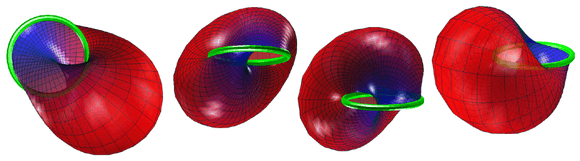The Sudanese Möbius band in the three-sphere S3 is geometrically a fibre bundle over a great circle, whose fibres are great semicircles. The most symmetrical image of a stereographic projection of this band into R3 is obtained by using a projection point that lies on that great circle that runs through the midpoint of each of the semicircles. Each choice of such a projection point results in an image that is congruent to any other. But because such a projection point lies on the Möbius band itself, two aspects of the image are significantly different from the case (illustrated above) where the point is not on the band: 1) the image in R3 is not the full Möbius band, but rather the band with one point removed (from its centerline); and 2) the image is unbounded – and as it gets increasingly far from the origin of R3, it increasingly approximates a plane. Yet this version of the stereographic image has a group of 4 symmetries in R3 (it is isomorphic to the Klein 4-group), as compared with the bounded version illustrated above having its group of symmetries the unique group of order 2. (If all symmetries and not just orientation-preserving isometries of R3 are allowed, the numbers of symmetries in each case doubles.)

But the most geometrically symmetrical version of all is the original Sudanese Möbius band in the three-sphere S3, where its full group of symmetries is isomorphic to the Lie group O(2). Having an infinite cardinality (that of the continuum), this is far larger than the symmetry group of any possible embedding of the Möbius band in R3.

A closely related 'strange' geometrical object is the Klein bottle. A Klein bottle can be produced by gluing two Möbius strips together along their edges; this cannot be done in ordinary three-dimensional Euclidean space without creating self-intersections.

Another closely related manifold is the real projective plane. If a circular disk is cut out of the real projective plane, what is left is a Möbius strip. Going in the other direction, if one glues a disk to a Möbius strip by identifying their boundaries, the result is the projective plane. To visualize this, it is helpful to deform the Möbius strip so that its boundary is an ordinary circle (see above). The real projective plane, like the Klein bottle, cannot be embedded in three-dimensions without self-intersections.

In graph theory, the Möbius ladder is a cubic graph closely related to the Möbius strip.

In 1968, Gonzalo Vélez Jahn (UCV, Caracas, Venezuela) discovered three dimensional bodies with Möbian characteristics, later described by Martin Gardner as prismatic rings that became toroidal polyhedrons.

## Applications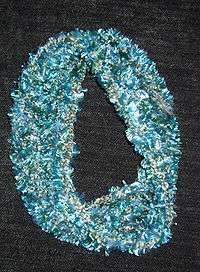Mathematical art: a scarf designed as a Möbius strip

There have been several technical applications for the Möbius strip. Giant Möbius strips have been used as conveyor belts that last longer because the entire surface area of the belt gets the same amount of wear, and as continuous-loop recording tapes (to double the playing time). Möbius strips are common in the manufacture of fabric computer printer and typewriter ribbons, as they let the ribbon be twice as wide as the print head while using both halves evenly.

A Möbius resistor is an electronic circuit element that cancels its own inductive reactance. Nikola Tesla patented similar technology in 1894: "Coil for Electro Magnets" was intended for use with his system of global transmission of electricity without wires.

The Möbius strip is the configuration space of two unordered points on a circle. Consequently, in music theory, the space of all two-note chords, known as dyads, takes the shape of a Möbius strip; this and generalizations to more points is a significant application of orbifolds to music theory.

In physics/electro-technology as:

• A compact resonator with a resonance frequency that is half that of identically constructed linear coils
• An inductionless resistor
• Superconductors with high transition temperature

In chemistry/nano-technology as:

• Molecular knots with special characteristics (Knotane , Chirality)
• Molecular engines
• Graphene volume (nano-graphite) with new electronic characteristics, like helical magnetism
• A special type of aromaticity: Möbius aromaticity
• Charged particles caught in the magnetic field of the earth that can move on a Möbius band
• The cyclotide (cyclic protein) kalata B1, active substance of the plant Oldenlandia affinis, contains Möbius topology for the peptide backbone.

1. Clifford A. Pickover (March 2005). The Möbius Strip : Dr. August Möbius's Marvelous Band in Mathematics, Games, Literature, Art, Technology, and Cosmology. Thunder's Mouth Press. ISBN 1-56025-826-8.
2. Rainer Herges (2005). Möbius, Escher, Bach – Das unendliche Band in Kunst und Wissenschaft . In: Naturwissenschaftliche Rundschau 6/58/2005. pp. 301–310. ISSN 0028-1050.
3. Chris Rodley (ed.) (1997). Lynch on Lynch. London, Boston. p. 231.
4. Starostin E.L.; van der Heijden G.H.M. (2007). "The shape of a Möbius strip". Nature Materials. 6 (8): 563–7. doi:10.1038/nmat1929. PMID 17632519.
5. Barr, Stephen (1964). Experiments in Topology. New York: Thomas Y. Crowell Company. pp. 48,200–201.
6. Dmitry Fuchs and Serge Tabachnikov, Mathematical Omnibus: Thirty Lectures on Classic Mathematics, 2007, page 199, at http://www.math.psu.edu/tabachni/Books/taba.pdf
7. Tony Phillips, Tony Phillips' Take on Math in the Media, American Mathematical Society, October 2006
8. Parker, Phillip (1993). "Spaces of Geodesics". Aportaciones Matemáticas. Notas de Investigación. UASLP: 67 − 79.
9. Hilbert, David; Cohn-Vossen, Stephan (1952). Geometry and the Imagination (2nd ed.). Chelsea. ISBN 0-8284-1087-9.
10. Dan Asimov; Doug Lerner (1984). "Issue 17 SIGGRAPH '84 Electronic Theater".
11. Spivak, Michael (1979). A Comprehensive Introduction to Differential Geometry, Volume I (2nd ed.). Wilmington, Delaware: Publish or Perish. p. 591.
12. Hilbert, David; Cohn-Vossen, S. (1999). Geometry and the Imagination (2nd ed.). Providence, Rhode Island: American Mathematical Society. p. 316. ISBN 978-0-8218-1998-2.
13. Gardner, Martin (1978). Mathematical Games. Providence, Rhode Island: Scientific American. pp. 12–13.
14. U.S. Patent 512,340
15. Clara Moskowitz, Music Reduced to Beautiful Math, LiveScience
16. Dmitri Tymoczko (7 July 2006). "The Geometry of Musical Chords". Science. 313 (5783): 72–4. doi:10.1126/science.1126287. PMID 16825563.
17. IEEE of Trans. Microwave Theory and Tech., volume. 48, No. 12, pp. 2465−2471, Dec. 2000
18. U.S. Patent 3,267,406
19. Enriquez, Raul Perez (2002). "A Structural parameter for High Tc Superconductivity from an Octahedral Moebius Strip in RBaCuO: 123 type of perovskite". Rev Mex Fis. 48 (supplement 1): 262. arXiv:cond-mat/0308019.
20. Angew Chem Int OD English one 2005 February 25; 44 (10): 1456−77.
21. Yamashiro, Atsushi; Shimoi, Yukihiro; Harigaya, Kikuo; Wakabayashi, Katsunori (2004). "Novel Electronic States in Graphene Ribbons -Competing Spin and Charge Orders-". Physica E. 22 (1–3): 688–691. arXiv:cond-mat/0309636. doi:10.1016/j.physe.2003.12.100.
22. IEEE Transactions on plasma Science, volume. 30, No. 1, February 2002Look up Möbius strip in Wiktionary, the free dictionary.Wikimedia Commons has media related to Möbius strip.